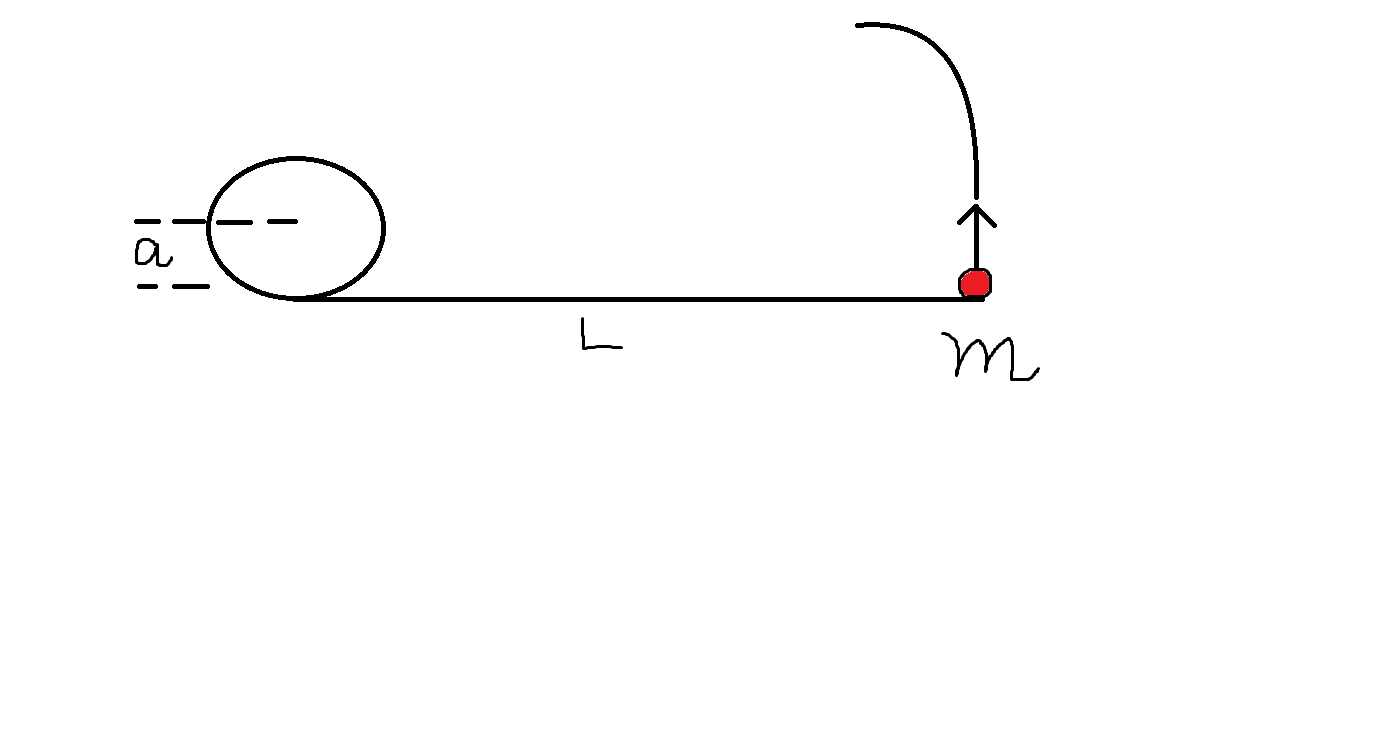# These springs again!A mass m is attached to one end of a spring of zero equilibrium length,the other end of which is fixed.The spring constant is K.Initial conditions are set up so that the mass moves around in a circle of radius L on a friction less horizontal table.

(By "zero equilibrium length" ,we mean that the equilibrium length is negligible compared to L)

At a given time ,a vertical pole(of radius a,with a<<L) is fixed onto the table next to the centre of the circle as in figure.The spring winds around,and the mass eventually hits the pole.Assume that any part of the spring touching the pole does not slip.The time taken for the mass to hit the pole is:-

$T=\frac { \Delta }{ \Delta +1 } \frac { L }{ a } \sqrt { \frac { m }{ K } }$

Find value of $\Delta$

PS:: I found this on a book and i couldn't solve it,if anyone can add a solution to this , that would be very kind of you.

×